## Automatic Sample-Time Interpolation at Input Port

This example shows how to directly use baseband communication signals using a lower sample rate in wider-band circuit envelope simulation. You will see how to perform an RF simulation using input signals with sample rate different from the simulation time step specified in the Configuration block.

The example requires Communications Toolbox™.

### Part 1: Single signal entering the RF system

The following model includes a Zigbee (802.15) baseband signal fed into a direct conversion RF receiver. The ZigBee baseband transmitter is built using blocks from Communications Toolbox and DSP System Toolbox™, while the RF receiver is modeled using RF Blockset.

For the RF Blockset Circuit Envelope solver it is recommended to use a simulation time step that is smaller than the reciprocal of the data rate of the input baseband signal. This provides a simulation bandwidth that is sufficient for the RF solver to capture artefacts at the edge of the bandwidth accurately and the physical effects that require additional bandwidth such as spectral regrowth. In general, using an interpolation factor of 4 to 8 allows increasing the simulation bandwidth beyond the Nyquist rate of the baseband signal generated in the transmitter. This example discusses the details of how to manage the rate transition correctly.

In the example, two different rates are shown in the model using different signal colors:

• green for the baseband signal sample rate

• red for the RF circuit envelope solver time step

```model = 'simrfV2_sampletime_example'; open_system(model); sim(model); % Do not show any scope results yet (see PostLoadFcn Model Callback % for more details): SpTxScopeConf.Visible = false; SpTXiScopeConf.Visible = false; SpRxScopeConf.Visible = false; ```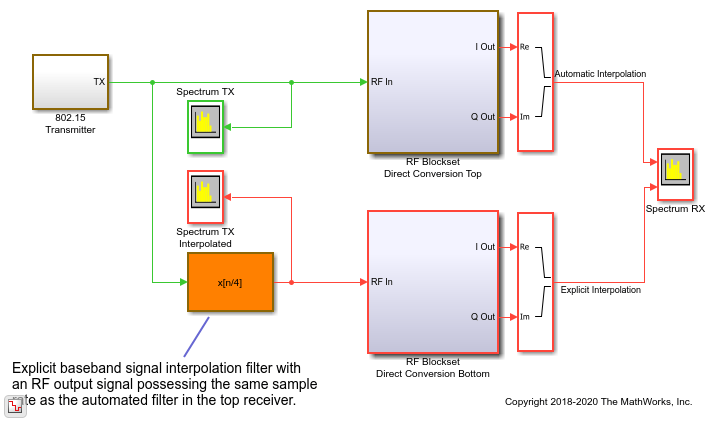The RF receiver systems in the model are identical and consists of Pre-LNA filter, followed by an LNA, quadrature demodulator, and another amplification stage. All RF components include typical impairments such as noise, nonlinearity, and finite isolation.

```open_system([model '/RF Blockset Direct Conversion RX1']); ```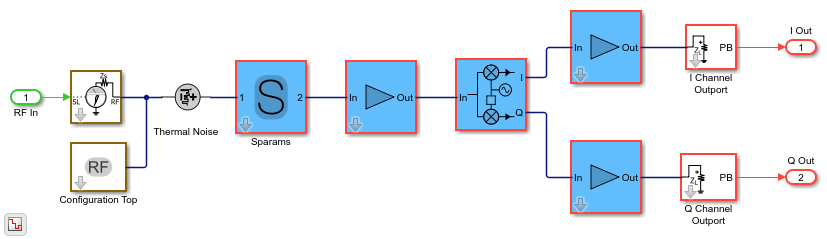As specified in the Configuration block Mask Parameters dialog box, the simulation is performed with input interpolation filter enabled for the top model, and disabled for the bottom model.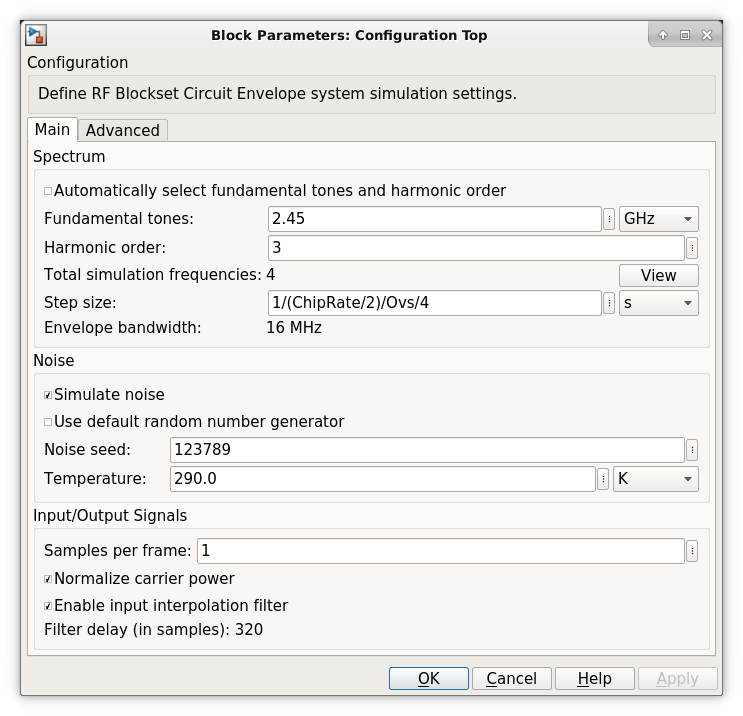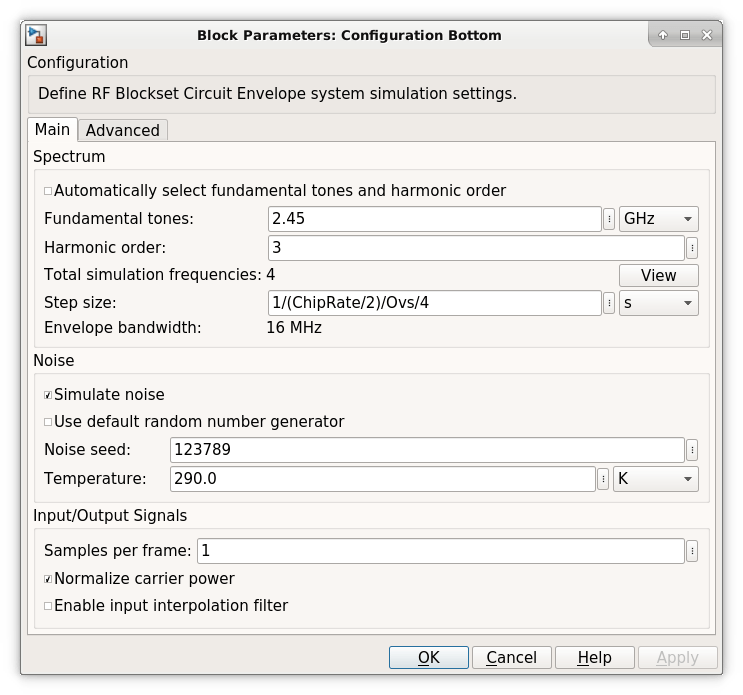The RF receiver on top is fed with a baseband signal with a sample rate that is 4 times slower than the rate used for RF simulation as set in the Configuration block. The model automatically interpolates the input signal to the desired rate.

The RF receiver at the bottom is fed with a baseband signal using a sample rate that is the same as the one set in the Configuration block and used for RF simulation. The model uses an explicit interpolation filter (highlighted in orange) in the model.

```% Show these two scope results: SpTxScopeConf.Visible = true; SpTXiScopeConf.Visible = true; ```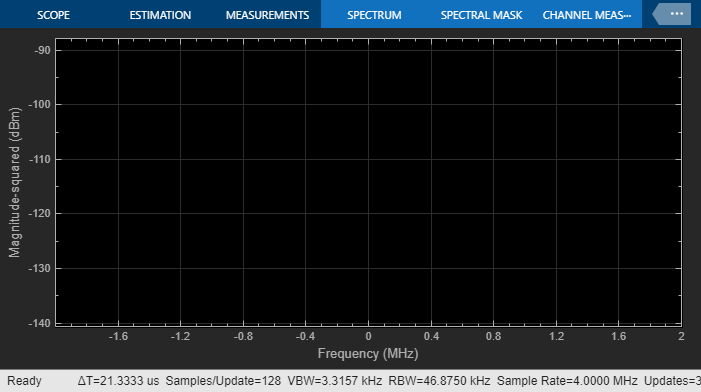The output of both receivers is the same and correct, as the input signal is resampled by an interpolation filter that reduces the aliasing effects due to the sample rate transition. In the top model, the sample rate transition is automatically managed by the circuit envelope configuration. In the bottom model, the sample rate transition is explicitly managed by the interpolation filter.

```% Show this scope result: SpRxScopeConf.Visible = true; ```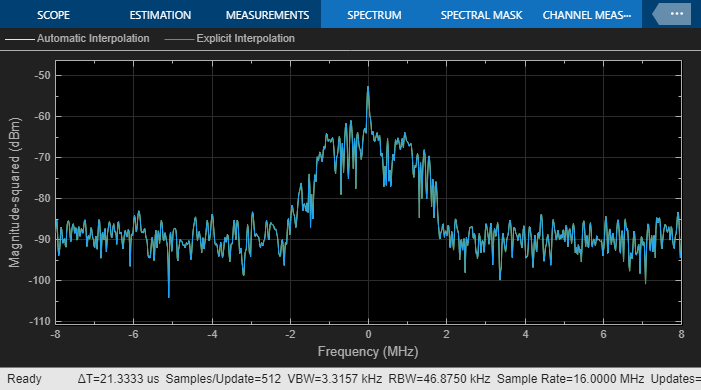Using an interpolation filter improves the spectral results of the simulation, but comes at a price: it introduces a delay. Since an FIR filter is used for the interpolation, the delay corresponds to half the number of filter coefficients. In this case, the filter has 640 taps. Therefore the interpolation filter introduces a delay of 320 time steps at the faster sample rate or 80 time steps at the slower sample rate. In case of multiple inputs, it might be necessary to compensate for this delay by aligning all signals entering the RF system.

When enabling the input interpolation filter at the bottom of the Configuration block Mask Parameters dialog box, the delay introduced by the interpolation filter at the faster sample rate of the RF solver is displayed in the Mask Parameters dialog box.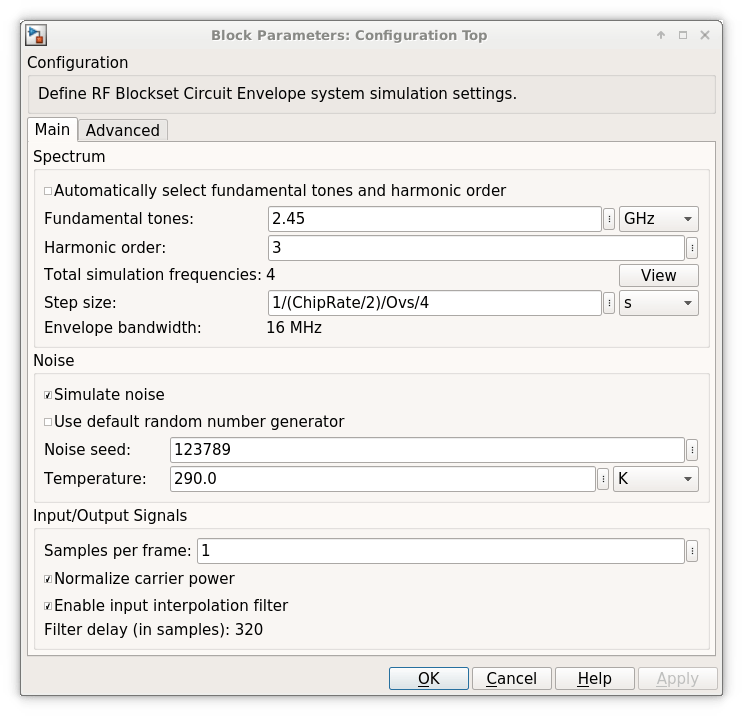By default RF Blockset automatically resamples the input signal and inserts an interpolation filter. You might want to decide to disable the default option and explicitly insert an interpolation filter if you have:

• specific requirements regarding the specs of the interpolation filter;

• multiple input signals requiring different input ports (case described below);

• Simulink control signals (e.g. applied to VGA, variable phase shifter, switch blocks) that are intrinsically slower than the RF signal and do not require resampling.

### Part 2: Multiple signals entering the RF system

RF Blockset supports general system topologies with multiple input ports. The automatic interpolation option discussed above is only supported for a single input port. When using multiple input ports the user is required to explicitly interpolate the signals to have them all enter the RF system with the sample rate defined in the RF Configuration block.

While RF Blockset input port accepts a vector of multiple signals each specified at a different carrier frequency, these signals must have the same sample rates. The following model describes two RF systems with multiple inputs centered around different carriers and correctly resampled. The model is like the one in Part 1 of this demo, but it also includes a wideband interfering signal that is generated using blocks from Communications Toolbox and DSP System Toolbox™. The two input signals have the same sample rate and the RF Blockset solver is faster by a factor of 4.

```bdclose(model); model = 'simrfV2_sampletime_example_interf1'; open_system(model); sim(model); ```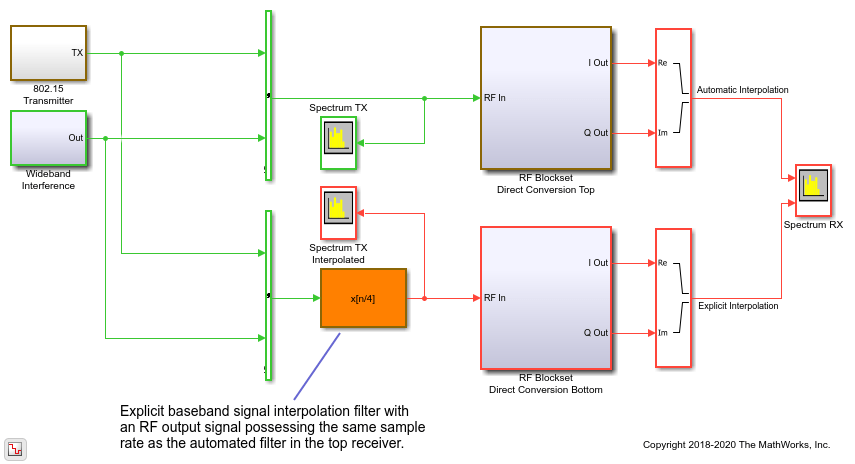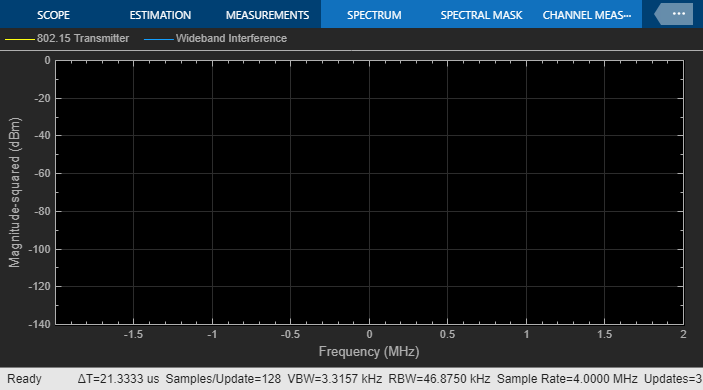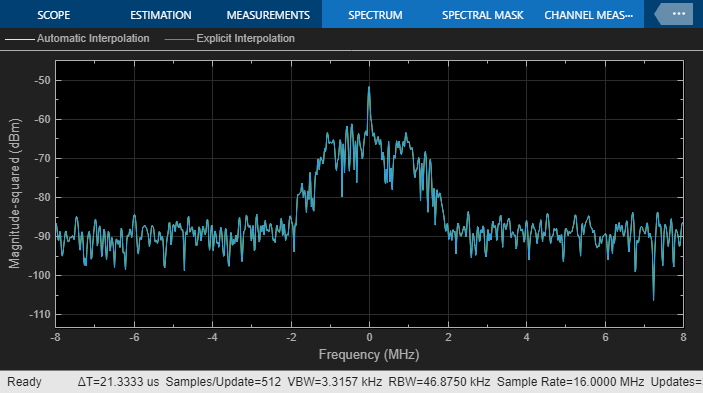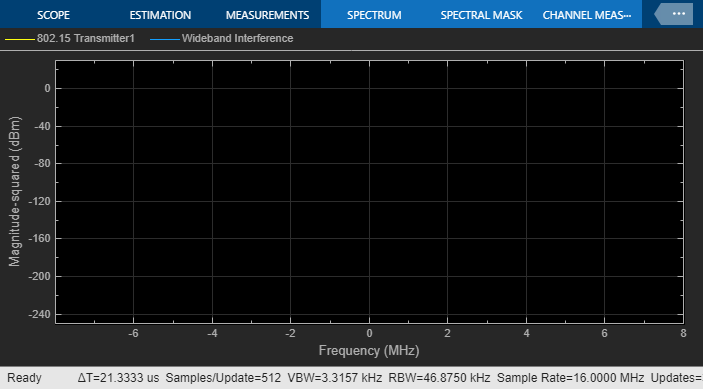The model is like the one described in Part 1 of this demo. Also in this case, interpolation is needed to avoid aliasing effects due to rate transition.

A more interesting scenario occurs when the desired and interferer signals have different sample rates. This can be seen in the following model. In this model, the desired signal is explicitly interpolated by the filter (highlighted in orange) before being combined in a vector together with the wideband interferer.

To avoid the aliasing effect, the slower rate of the desired input signal must be interpolated and filtered before being combining with the interfering signal at a faster rate.

```bdclose(model); model = 'simrfV2_sampletime_example_interf2'; open_system(model); sim(model); % Do not show any scope results yet (see PostLoadFcn Model Callback % for more details): SpTXComScopeConf.Visible = false; SpRxSepScopeConf.Visible = false; SpRxComScopeConf.Visible = false; ```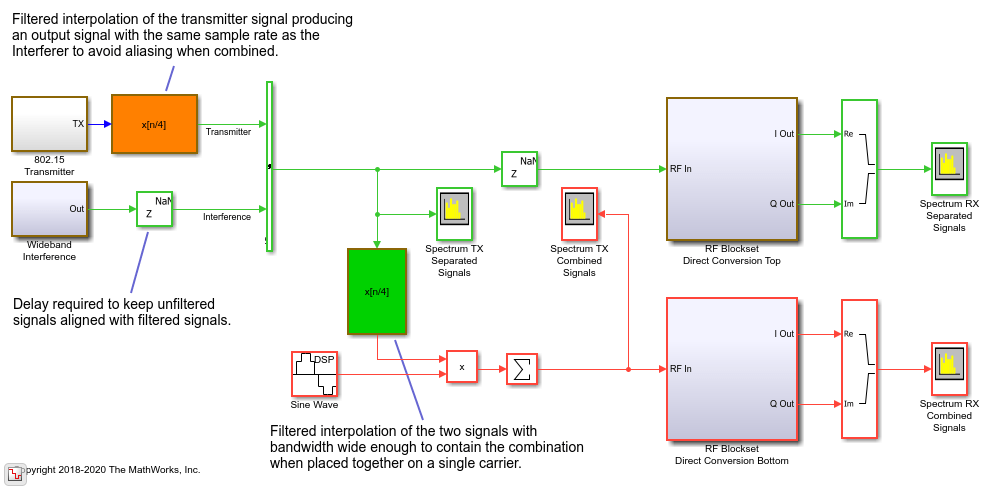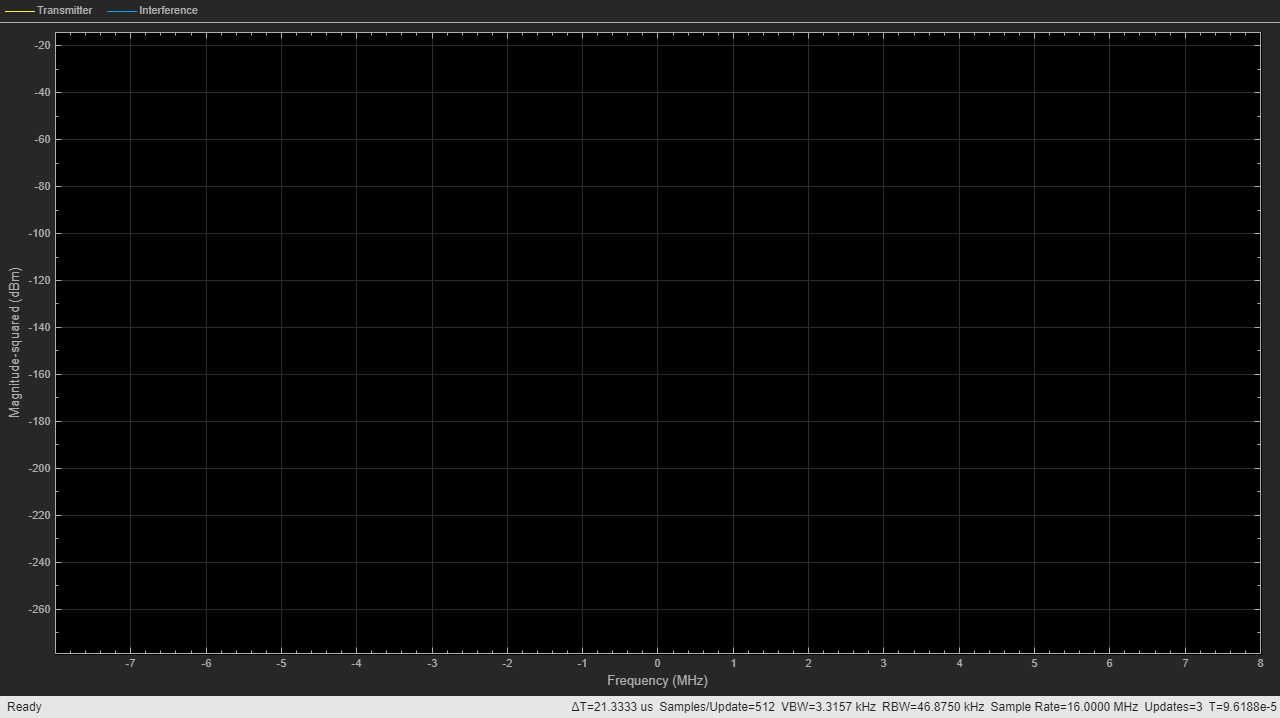In the top RF model, the two signals are entering the RF system centered around two different carriers. Note that the sample rate of the signal entering the top RF system is already the same as the one defined in the RF Configuration block. Therefore, enabling the automatic input interpolation filter in the RF Configuration block in this case does not introduce any interpolation.

```SpRxSepScopeConf.Visible = true; ```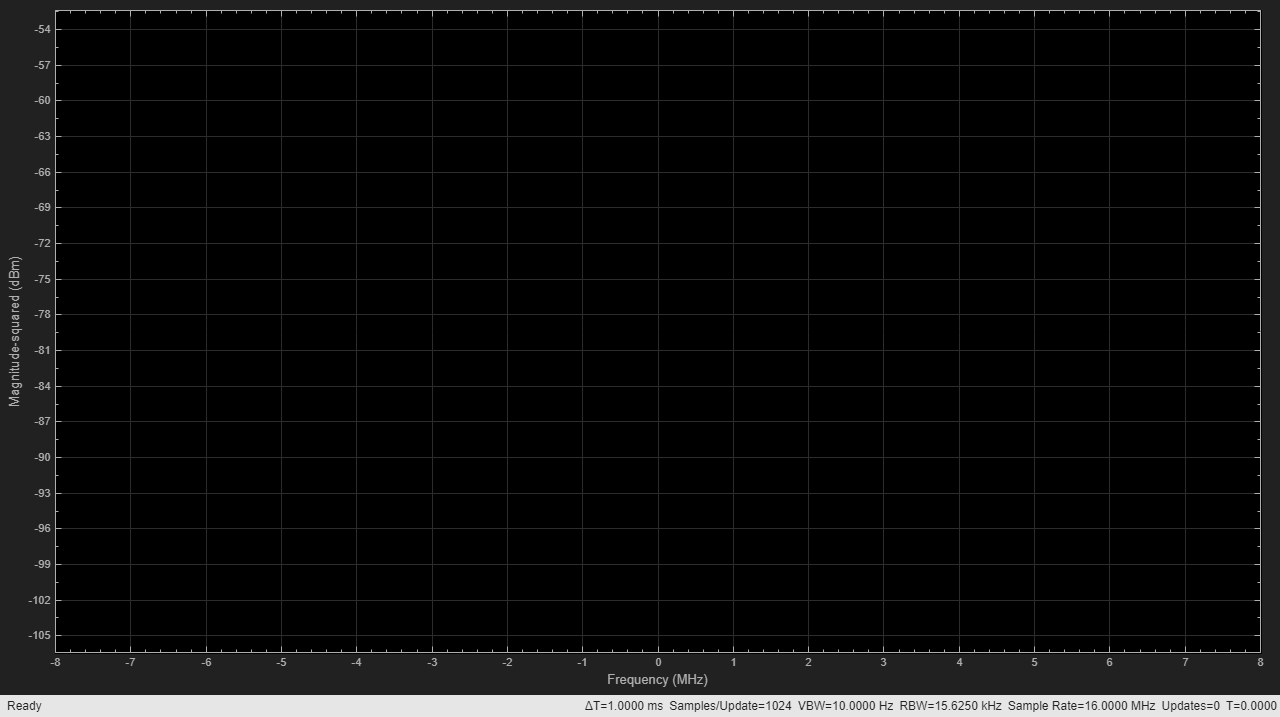The last scenario discussed in this demo occurs when the two signals entering the RF system are placed on carriers that are relatively close to each other. Since the number of mixing harmonics that need to be simulated can be large if the system exhibits strong nonlinearity, it is recommended to combine the two signals into one carrier when they are close by.

```SpTXComScopeConf.Visible = true; ```In the bottom model, the lower RF system is fed by the desired and interfering signals combined in a single envelope. The combined signal is achieved by the multiplication of the interferer signal with a complex exponent that shifts its operation frequency by 20MHz relative to the frequency of the desired signal. Note that the bandwidth needed to capture both signals when combined on a single carrier is larger than the bandwidth of each individual signal. This is the reason for introducing the interpolation filter highlighted in green before combining the signals.

```SpRxComScopeConf.Visible = true; ```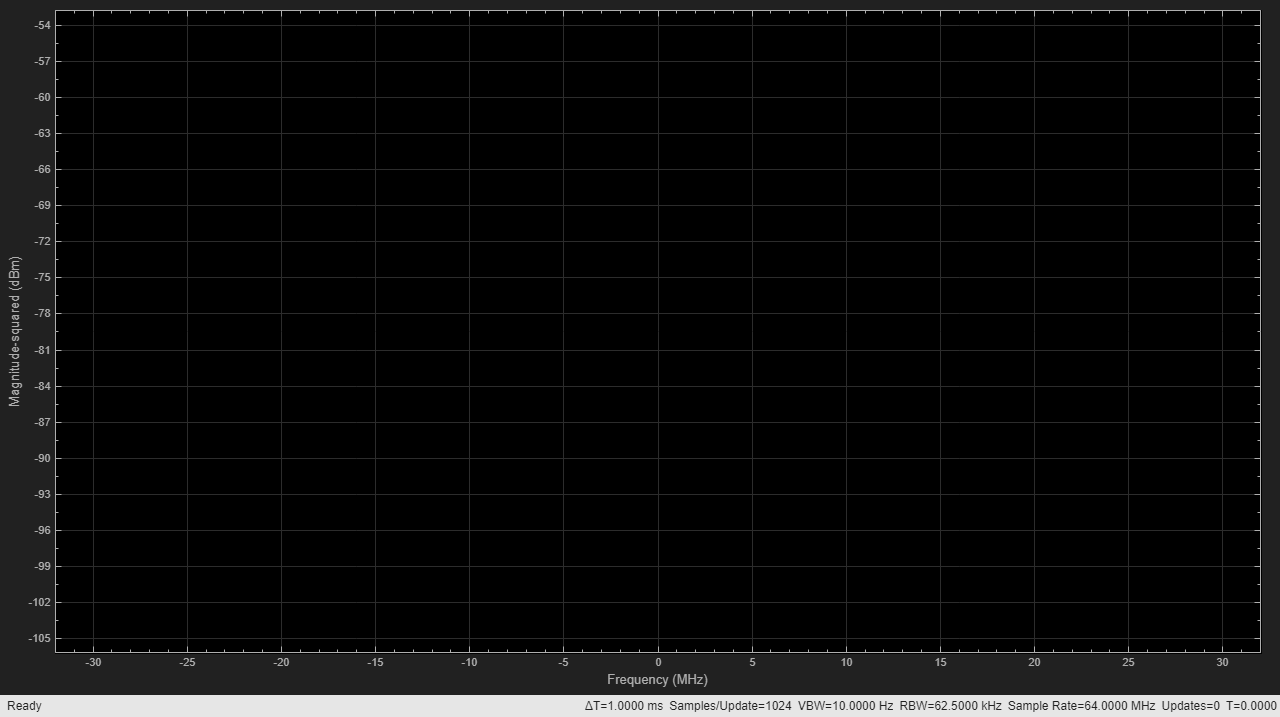The results of the two RF systems (top and bottom) in the above model shows excellent correspondence. The interferer signal is missing from the spectrum in the upper RF system since the output port behaves as an ideal filter and only selects the real passband signal centered at DC. The interferer signal is missing from the spectrum in the lower RF system since the IQ Demodulator includes a channel select filter. To see the effects of the interferer signal, you can turn off the filter by unchecking the 'Add Channel Select filter' checkbox in the IQ Demodulator block Mask Parameter dialog. The resulting spectrum is seen below.

```set_param([model '/RF Blockset Direct Conversion RX2/IQ Demodulator'], ... 'AddCSFilters', 'off'); sim(model); % Do not show other scopes and rescale Y axis: SpTxSepScopeConf.Visible = false; SpTXComScopeConf.Visible = false; SpRxSepScopeConf.Visible = false; SpRxComScopeConf.YLimits = [-103 0]; ```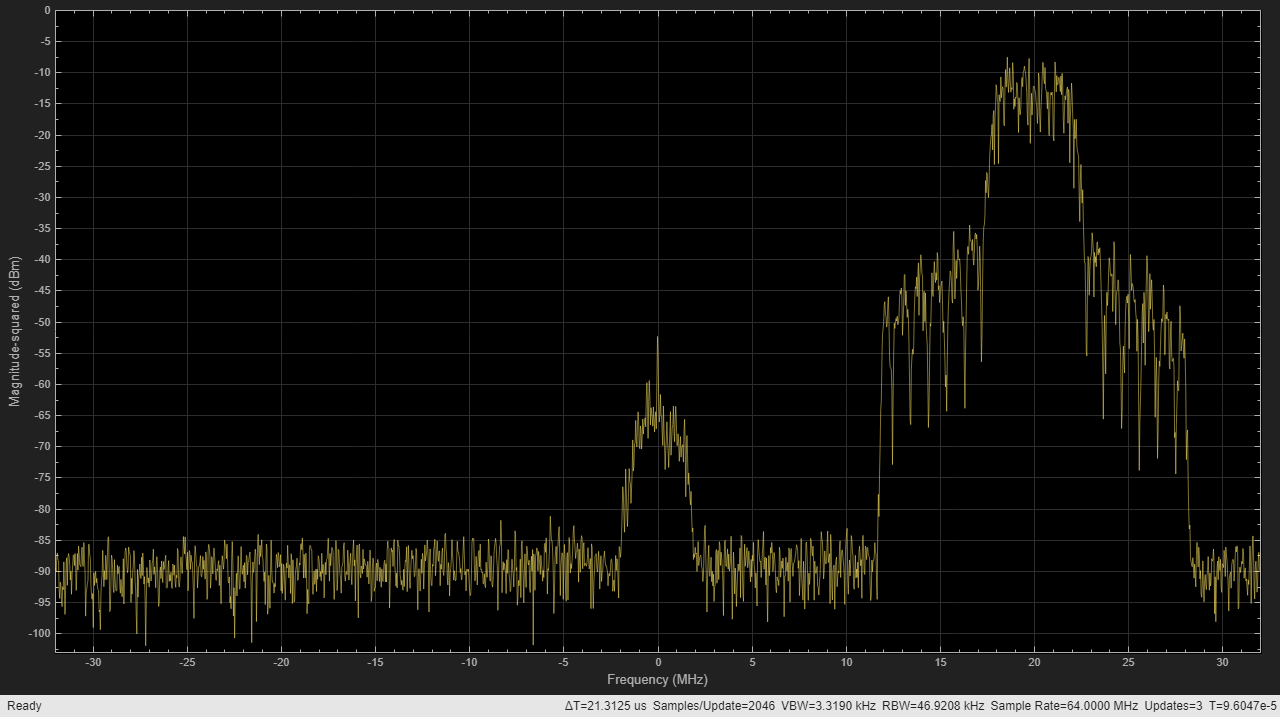```bdclose(model); clear model; ```